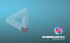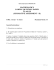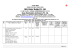# Class 9 - Sample Question Paper (Mathematics) I – SA-I

## Transcription

Class 9 - Sample Question Paper (Mathematics) I – SA-I
```Class 9 - Sample Question Paper (Mathematics) I – SA-I
Time allowed: 3 hours Maximum marks: 80
General Instructions:
(i)
All questions are compulsory.
(ii)
The question paper consists of 34 questions divided into four sections – A, B, C
and D.
(iii) Section A contains 10 questions of 1 mark each, which are multiple choice type
questions, section B contains 8 questions of 2 marks each, section C contains 10
questions of 3 marks each and section D contains 6 questions of 4 marks each.
(iv) There is no overall choice in the paper.
(v)
Use of calculators is not permitted.
Section – A
Question numbers from 1 to 10 are of one mark each.
1. Decimal expansion of a rational number can never be
a) Terminating
b) Non-terminating
c) Non-terminating non-recurring
d) Non-terminating recurring
2. Which of the following is a rational number between 3 and 4?
a) √
b) .010010001…
c)
d)
3. The degree of the zero polynomial is
a) 0
b) 1
c) 1
d) Not defined
Page 1
4. If
a) 0
b) 1
c)
d)
0 then
5. In which quadrant the coordinates are of the form
a)
b)
c)
d)
6. Number of straight lines that can be drawn through two given points
a) 1
b) 2
c) 0
d) Infinite
7. If three or more points lie on the same line then they are called as
a) Coplanar points
b) Collinear points
c) Concurrent points
d) Non-collinear points
8. In the figure shown
a)
b)
c)
d)
, then
9. Two sides of a triangle are 6.5 cm and 5 cm. The length of the third side cannot be
a) 10.5 cm
b) 11 cm
c) 1.5 cm
d) 9.5 cm
Page 2
10. In
and
triangles are congruent by which axiom?
a) SAS congruence axiom
b) ASA congruence axiom
c) RHS congruence axiom
d) SSS congruence axiom
and
then the two
Section – B
Question numbers 11 to 18 carry 2 marks each.
11. Find 4 rational numbers between and .
12. Rationalize the denominator of
13. Factorize:
√
√
1
14. Find the remainder when
10 is divided by (
).
15. Write the coordinates of P, Q, R and S shown in the following graph.
16. In the figure shown. AB||CD. Find
.
17. In an isosceles triangle ABC with AB = AC, D and E are points on BC such that BE =
CD. Show that AD = AE.
18. Show that in a right angled triangle, the hypotenuse is the longest side.
Page 3
Section – C
Question numbers 19 to 28 carry 3 marks each.
19. Evaluate . ̅̅̅̅ 0.1 ̅
20. Define (i) Radius (ii) Parallel lines (iii) Perpendicular lines (iv) Line segment.
21. Plot the following points:
1
2
4
5
22. A p rk i the sh pe of qu ril ter l ABCD h s C
CD = 5 m and AD = 8 m. How much area does it occupy?
0 , AB = 9 m, BC = 12 m,
23. If the polynomial
11 is divided by
leaves a
remainder
. Find the value of .
24. Divide
11
1 by
1.
25. Prove th t “if tr svers l i tersects two p r llel li es the e ch p ir of lter te
i terior gles is equ l”.
26. If a transversal intersects two lines such that the bisectors of a pair of
corresponding angles are parallel, then prove that the two lines are parallel.
27. Angles opposite to equal sides of an isosceles triangle are equal – prove.
28. D is poi t o si e BC of ABC such th t AD AC. Show th t AB > AD.
Page 4
Section – D
Question numbers 29 to 34 carry 4 marks each.
29. Represent √ on number line.
30. Verify
31. AB is a line-segment. P and Q are points on opposite sides of AB such that each of
them is equidistant from the points A and B. Show that the line PQ is the
perpendicular bisector of AB.
32. Prove that the sum of the angles in a triangle is 1 0 .
33. In figure show the si es AB
AC of ABC re pro uce to poi ts E
D
respectively. If bisectors BO
CO of CBE
BCD respectively meet t poi t
O, then prove that
0
Page 5
34.
ABC is
isosceles tri gle i which AB
AD AB. Show th t BCD is right gle.
AC. Si e BA is pro uce to D such th t
www.learnnext.com/sample-papers.htm
Page 6
Page 7
```

### A golden opportunity to A golden opportunity to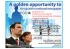### Class 10 - Sample Question Paper (Mathematics) II – SA-I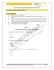### Class 10 - Sample Question Paper (Mathematics) I – SA-I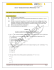### Our Brochure: HRbuz 2015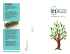### Security Settings For Windows 7 and Above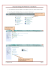### Sample Test Paper – 20 Marks Auditing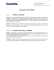### Document 6537741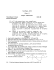### Dear Customer, HP India promotes its wide range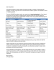### Imperium Technology Solutions (P) Ltd.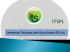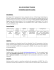### Final New Corporate overview - PRINTING.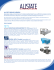### 2013 dt 04.07.2013 on th - the official website of Bangalore Customs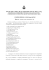### Summit Agenda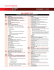### Stall Booking Form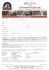### Summative Assessment-I Topper Sample Paper - 8 MATHEMATICS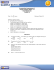### Summative Assessment-I Topper Sample Paper - 3 MATHEMATICS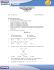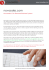### Catalog - Zeus Engitech Pvt. Ltd.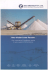### 2011 - The Couchiching Conservancy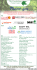### Shreenathji Worldwide Pvt. Ltd.